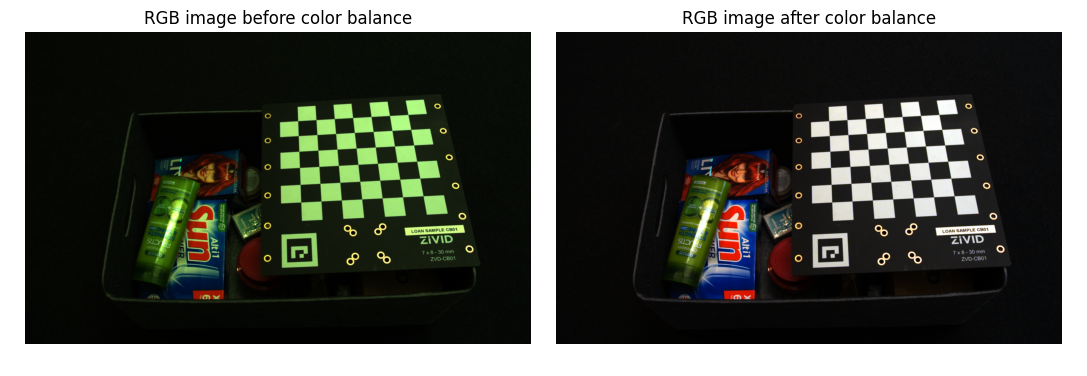# Color Balance

This tutorial shows how to balance the color of a 2D image by taking images of a white surface (a piece of paper, wall, or similar) in a loop.First, we connect to the camera.

```camera = app.connect_camera()
```

Then we automatically configure 2D acquisition settings by taking images in a loop while tuning gain, exposure time, and aperture. The goal is for the maximum of mean RGB values to reach the value within defined limits.

```settings_2d = _auto_settings_configuration(camera)
```

We then capture an image and display it.

```rgba = camera.capture(settings_2d).image_rgba().copy_data()
display_rgb(rgba[:, :, 0:3], title="RGB image before color balance", block=False)
```

Then we run the calibration algorithm, which balances the color of RGB image by taking images of a white surface (a piece of paper, wall, or similar) in a loop.

```[red_balance, green_balance, blue_balance] = _color_balance_calibration(camera, settings_2d)
```

The complete implementation of the algorithm is given below.

```def _color_balance_calibration(camera, settings_2d):
"""Balance color for RGB image by taking images of white surface (piece of paper, wall, etc.) in a loop.

Args:
camera: Zivid camera
settings_2d: 2D capture settings

Returns:
corrected_red_balance: Corrected red balance
corrected_green_balance: Corrected green balance
corrected_blue_balance: Corrected blue balance

"""
print("Starting color balance calibration")

corrected_red_balance = 1.0
corrected_green_balance = 1.0
corrected_blue_balance = 1.0

saturated = False

while True:
settings_2d.processing.color.balance.red = corrected_red_balance
settings_2d.processing.color.balance.green = corrected_green_balance
settings_2d.processing.color.balance.blue = corrected_blue_balance
rgba = camera.capture(settings_2d).image_rgba().copy_data()
mean_color = _compute_mean_rgb(rgba[:, :, 0:3], 100)
print(" Mean color values:")
print(f"  R: {int(mean_color.red)}")
print(f"  G: {int(mean_color.green)}")
print(f"  B: {int(mean_color.blue)}")
if int(mean_color.green) == int(mean_color.red) and int(mean_color.green) == int(mean_color.blue):
print("Color balance successful")
break
if saturated is True:
print("Color balance incomplete - the range limits of color balance parameters have been reached")
break
max_color = max(mean_color.red, mean_color.green, mean_color.blue)
corrected_red_balance = np.clip(settings_2d.processing.color.balance.red * max_color / mean_color.red, 1, 8)
corrected_green_balance = np.clip(
settings_2d.processing.color.balance.green * max_color / mean_color.green, 1, 8
)
corrected_blue_balance = np.clip(settings_2d.processing.color.balance.blue * max_color / mean_color.blue, 1, 8)

corrected_values = [corrected_red_balance, corrected_green_balance, corrected_blue_balance]

if 1.0 in corrected_values or 8.0 in corrected_values:
saturated = True
print("Color balance:")
print(f" Red: {corrected_red_balance}")
print(f" Green: {corrected_green_balance}")
print(f" Blue: {corrected_blue_balance}")

return (corrected_red_balance, corrected_green_balance, corrected_blue_balance)

```

Lastly, we apply the color balance settings, capture a new image and display it.

```settings_2d.processing.color.balance.red = red_balance
settings_2d.processing.color.balance.green = green_balance
settings_2d.processing.color.balance.blue = blue_balance
rgba_balanced = camera.capture(settings_2d).image_rgba().copy_data()
display_rgb(rgba_balanced[:, :, 0:3], title="RGB image after color balance", block=True)
```

The figure below shows the color image before and after applying color balance.